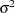Displayed Output

PROC ORTHOREG displays the parameter estimates and associated statistics. These include the following:

• overall model analysis of variance, including the error mean square, which is an estimate of(the variance of the true errors), and the overall F test for a model effect.

• root mean square error, which is an estimate of the standard deviation of the true errors. It is calculated as the square root of the mean squared error.

• R square () measures how much variation in the dependent variable can be accounted for by the model. R square, which can range from 0 to 1, is the ratio of the sum of squares for the model to the corrected total sum of squares. In general, the larger the value of R square, the better the model’s fit.

• estimates for the parameters in the linear model

The table of parameter estimates consists of the following:

• the terms used as regressors, including the intercept.

• degrees of freedom (DF) for the variable. There is one degree of freedom for each parameter being estimated unless the model is not full rank.

• estimated linear coefficients.

• estimates of the standard errors of the parameter estimates.

• the criticalvalues for testing whether the parameters are zero. This is computed as the parameter estimate divided by its standard error.

• the two-sided-value for thetest, which is the probability that astatistic would obtain a greater absolute value than that observed given that the true parameter is zero.